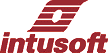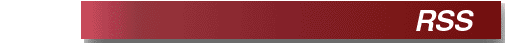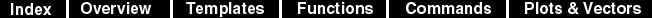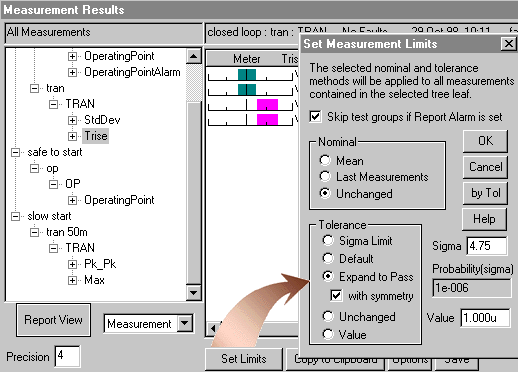RSS, Root Summed Square In this analysis (actual RSS.SCP file), after running a reference simulation, each parameter is perturbed and another analysis is run. For each measurement vector, the difference between the reference and the perturbed result is saved. This results in a number of simulations equal to the number of parameters that have tolerances. We then square the sensitivities of each measurement and sum them for each parameter. The square root of the result is saved in plot called "rss". Mathematically, the result for a single measurement is Vresult = sqrt( S ( Vresult(param) - Vresult(nominal) )^2 ) The RSS results are printed to the IsSpice4 output file in a format that can be read back in by SpiceNet. You can set the measurement test limits by expanding the measurements to "pass with symmetry" in the Results dialog; as shown below.If the result is linearly proportional to the change in the parameter value, then the RSS result is proportional to the standard deviation which we could obtain from a statistical analysis. As a matter of convention, each tolerance is taken as the 3 sigma value, or 3 standard deviations and we report the RSS results as though they were also 3 sigma. The parameter perturbation is set to 1 sigma, a compromise between a negligibly small value and the entire tolerance band. Making the perturbation fairly large eliminates some errors due to local maximum values occurring nearby. You can change this variation by editing the RSS Simulation Template. For many circuits, the variation of a measurement with respect to some parameters is highly nonlinear so that this analysis will give incorrect results, frequently reporting smaller measurement variations than would be found using a statistical analysis. When performing an AC analysis, it is frequently assumed that the response at each frequency can be considered independently. Unfortunately, this is a poor assumption because single frequency results have an ambiguity in phase. When the difference in phase between 2 simulations is taken, there can be dramatically different results if one analysis is in a different phase plane than the reference simulation. Because of this, it is necessary to phase extend the vectors we wish to measure. This makes the AC analysis similar to the transient analysis, requiring measurements that resolve to scalar values. The duality between frequency and time seen using the Fourier transform should make this obvious.